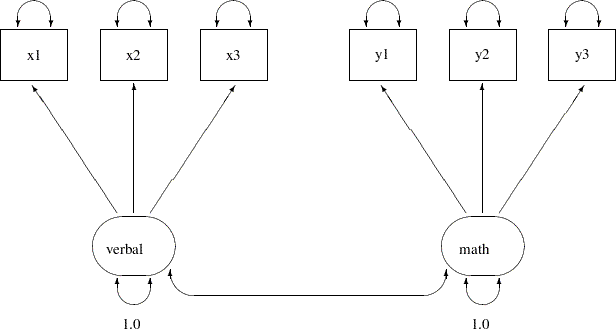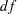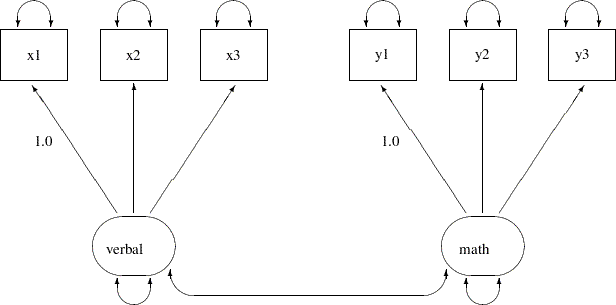Previous Page | Next Page

 The CALIS Procedure

## Example 25.11 Confirmatory Factor Models

This example shows how you can fit a confirmatory factor-analysis model by the FACTOR modeling language. Thirty-two students take tests of their verbal and math abilities. Six tests are administered separately. Tests x1x3 test their verbal skills and tests y1y3 test their math skills.

The data are shown in the following DATA step:

```data scores;
input x1 x2 x3 y1 y2 y3;
datalines;
23  17  16  15  14  16
29  26  23  22  18  19
14  21  17  15  16  18
20  18  17  18  21  19
25  26  22  26  21  26
26  19  15  16  17  17
14  17  19   4   6   7
12  17  18  14  16  13
25  19  22  22  20  20
7  12  15  10  11   8
29  24  30  14  13  16
28  24  29  19  19  21
12   9  10  18  19  18
11   8  12  15  16  16
20  14  15  24  23  16
26  25  21  24  23  24
20  16  19  22  21  20
14  19  15  17  19  23
14  20  13  24  26  25
29  24  24  21  20  18
26  28  26  28  26  23
20  23  24  22  23  22
23  24  20  23  22  18
14  18  17  13  16  14
28  34  27  25  21  21
17  12  10  14  12  16
8   1  13  14  15  14
22  19  19  13  11  14
18  21  18  15  18  19
12  12  10  13  13  16
22  14  20  20  18  19
29  21  22  13  17  12
;
```

Because of the unambiguous nature of the tests, you hypothesize that this is a confirmatory factor model with two factors: one is the verbal ability factor and the other is the math ability factor. You can represent such a confirmatory factor model by the following path diagram:In the path diagram, there are two clusters of variables. One cluster is for the verbal factor and the other is for the math factor. The single-headed arrows in the path diagram represent functional relationships between factors and the observed variables. The double-headed arrows that point to single variables represent variances of the factors or error variances of the observed variables. The double-headed arrow that connect the two factors represents their covariance. All but two of these arrows are not labeled with numbers. Each of the unlabeled arrows represents a free parameter in the confirmatory factor model. You label the double-headed arrows that attach to the two factors with the constant 1. This means that the variances of the factors are fixed at 1.0 in the model.

You can specify the confirmatory factor model by the FACTOR model language of PROC CALIS, as shown in the following statements:

```proc calis data=scores;
factor
verbal ---> x1-x3,
math   ---> y1-y3;
pvar
verbal = 1.,
math   = 1.;
run;
```

In each of the entry of the FACTOR statement, you specify a latent factor, followed by a list of observed variables that are functionally related to the latent factor. For example, in the first entry, the verbal factor is related to variables x1x3, as shown by the single-headed arrows in the path diagram. In fact, all single-headed arrows in the path digram are specified in the FACTOR statement. Notice that each entry of the FACTOR statement must take the format of

```factor_name ---> variable_list
```

You cannot reverse the arrow specification as in the following:

```variable_list <--- factor_name
```

Nor you can have a specification such as the following:

```variable_list ---> factor_name
```

However, you can specify the functional relationships between factors and variables in different entries. For example, you can specify the same confirmatory factor model by the following statements:

```title "Basic Confirmatory Factor Model: Separate Path Entries";
title2 "FACTOR Model Specification";
proc calis data=scores;
factor
verbal ---> x1,
verbal ---> x2,
verbal ---> x3,
math   ---> y1,
math   ---> y2,
math   ---> y3;
pvar
verbal = 1.,
math   = 1.;
fitindex  noindextype on(only)=[chisq df probchi rmsea  srmsr bentlercfi];
run;
```

In the PVAR statement, which is for the specification of variances or error variances, you fix the variances of the latent factors to 1. This completes the model specification of the confirmatory factor model, although you do not specify other arrows in the path diagram as free parameters in these statements. The reason is that in the FACTOR modeling language, the variances and covariances among factors and the error variances of the observed variables are default parameters in the confirmatory factor model. It is not necessary to specify these parameters (or the corresponding arrows in the path diagram) explicitly if they are free parameters in the model. You can also specify these free parameters explicitly without affecting the estimation. However, if these parameters (or the corresponding double-headed arrows in the path diagram) are intended to be constrained parameters or fixed values, you must specify them explicitly. For example, in the current confirmatory factor model, you must provide explicit specifications for the variances of the verbal and the math factors because these parameters are fixed at 1.

Output 25.11.1 shows the modeling information and the variables in the confirmatory factor model.

Output 25.11.1 Modeling Information and Variables of the CFA Model: Scores Data
 Simple Confirmatory Factor Model FACTOR Model Specification

The CALIS Procedure
Covariance Structure Analysis: Model and Initial Values

Modeling Information
Data Set WORK.SCORES
N Records Used 32
N Obs 32
Model Type FACTOR
Analysis Covariances

Variables in the Model
Variables x1 x2 x3 y1 y2 y3
Factors verbal math

In the beginning of the output, PROC CALIS shows the data set, the number of observations, the model type, and the analysis type. The default analysis type in PROC CALIS is covariances (that is, covariance structures). If you want to analyze the correlation structures instead, you can use the CORR option in the PROC CALIS statement. Next, PROC CALIS shows the list of variables and factors in the model. As expected, the number of variables is 6 and the number of factors is 2.

Output 25.11.2 shows the initial model specifications of the confirmatory factor model.

Output 25.11.2 Initial Specification of the CFA Model: Scores Data
verbal math
x1
 . [_Parm1]
 0
x2
 . [_Parm2]
 0
x3
 . [_Parm3]
 0
y1
 0
 . [_Parm4]
y2
 0
 . [_Parm5]
y3
 0
 . [_Parm6]

Initial Factor Covariance Matrix
verbal math
verbal
 1.0000
math
 1.0000

Initial Error Variances
Variable Parameter Estimate

The first table of Output 25.11.2 shows the pattern of factor loadings of the variables on the two latent factors. As expected, x1x3 have nonzero loadings only on the verbal factor, while y1y3 have nonzero loadings on the math factor. PROC CALIS names these free parameters automatically with the "_Parm" prefix and unique numerical suffixes. There are six parameters in the factor loading matrix with six different parameter names.

The next table of Output 25.11.2 shows the covariance matrix of the factors. The variances of the factors are fixed at one, as shown on the diagonal of the covariance matrix. The covariance between the two factors is a free parameter named _Add1. You did not specify this covariance parameter explicitly in the factor model specification. By default, PROC CALIS assumes that latent factors are correlated. Default free parameters added by PROC CALIS have the _Add prefix for their names. If you do not want to assume the covariances among the factors, you must specify zero covariances in the COV statement. For example, the following statement specifies that the math and verbal factors have zero covariance:

``` COV
math verbal = 0.;
```

The last table of Output 25.11.2 shows the error variance parameters of the observed variables. By default PROC CALIS assumes these error variances are free parameters in the confirmatory factor model. These added parameters are named with the _Add prefix. However, as all other default parameters that are assumed by PROC CALIS, you can overwrite the default by using explicit specifications. You can specify the error variances of a confirmatory factor model explicitly in the PVAR statement. See specifications in Example 25.12.

Output 25.11.3 shows the fit summary of the confirmatory factor model for the scores data.

Output 25.11.3 Fit Summary of the CFA Model: Scores Data
Fit Summary
Chi-Square 9.8052
Chi-Square DF 8
Pr > Chi-Square 0.2790
Standardized RMSR (SRMSR) 0.0571
RMSEA Estimate 0.0853
Bentler Comparative Fit Index 0.9887

The model fit chi-square is 9.805 (=8,=0.279). This shows that statistically you cannot reject the confirmatory factor model for the test scores. However, the root mean square error of approximation (RMSEA) estimate is 0.0853, which is larger than the conventional 0.05 value for a good model fit. The standardized root mean square residual (SRMSR) is 0.0571, which is close to the conventional 0.05 value for a good model fit. Bentler’s comparative fit index is 0.9887, which indicates a very good model fit. Overall, the model seems to be quite reasonable for the data.

Output 25.11.4 shows the loading and factor covariance estimates of the confirmatory factor model for the scores data. The first table shows the loading estimates, together with the standard error estimates and thevalues. In structural equation modeling, the significance of the parameter estimates is usually inferred by comparing thevalues with the critical value of a standardized normal variate (that is, the-table). Therefore, estimates with associated (absolute)values greater than 1.96 are significant at=.05. In Output 25.11.4, all thevalues for the loading estimates are greater than 2. This indicates that the prescribed relationships between the variables and the factors are significant.

Output 25.11.4 Loading and Factor Covariance Estimates of the CFA Model: Scores Data
verbal math
x1
 5.8406 0.9962 5.8629 [_Parm1]
 0
x2
 5.8182 0.9537 6.1004 [_Parm2]
 0
x3
 4.6619 0.7814 5.9662 [_Parm3]
 0
y1
 0
 5.2804 0.6998 7.5455 [_Parm4]
y2
 0
 4.2003 0.6220 6.7532 [_Parm5]
y3
 0
 3.7596 0.6341 5.9289 [_Parm6]

Factor Covariance Matrix: Estimate/StdErr/t-value
verbal math
verbal
 1.0000
math
 1.0000

The second table of Output 25.11.4 shows the covariance matrix of the verbal and the math factors. Because the factor variances are fixed at one, the covariance estimate is also the correlation between the two factors. Output 25.11.4 shows that the two factors are moderately correlated with a correlation estimate of 0.5175, which is statistically significant.

Output 25.11.5 shows the estimates of the error variances. All but the error variance of y1 are significant. This suggests that y1 might have an almost perfect relationship with the math factor.

Output 25.11.5 Error Variance Estimates of the CFA Model: Scores Data
Error Variances
Variable Parameter Estimate Standard
Error
t Value

Output 25.11.6 echoes this same fact. The R-squares in this table shows the percentages of variance of the variables that are overlapped with the factors. While all these percentages (0.74 – 0.97) are quite high for all variables, the percentage is especially high for y1. It shares 97% of the variance with the math factor. So, it appears that the observed variable y1 is almost a perfect indicator of the math factor.

Output 25.11.6 Squared Multiple Correlations of the CFA Model: Scores Data
Squared Multiple Correlations
Variable Error Variance Total Variance R-Square
x1 11.52376 45.63609 0.7475
x2 9.14503 42.99597 0.7873
x3 6.68169 28.41532 0.7649
y1 0.78580 28.66835 0.9726
y2 2.88069 20.52319 0.8596
y3 5.15573 19.29032 0.7327

#### Alternative Identification Constraints

Setting the variances of the latent factors to 1 in the preceding FACTOR model specification makes the model identified. This is necessary because the scales of the latent factors are arbitrary and the constraints imposed on the factor variances fix the scales of the factors.

In practice, there is another way to fix the scales of the factors. For each factor, you can fix the loading of one of its measured indicators to a constant. This fixed loading value is usually set at 1. For example, you can represent the confirmatory factor model for the scores data by the following alternative path diagram:This path diagram is essentially the same as the preceding one. However, the fixed constants adjacent to the double-headed arrows that attach to the two factors in the preceding path diagram are now moved to two of the single-headed paths in the current path diagram.

You can specify this path diagram by the following FACTOR model specification of PROC CALIS:

```proc calis data=scores;
factor
verbal ---> x1-x3   = 1. ,
math   ---> y1-y3   = 1. ;
run;
```

In the FACTOR statement, you assign a fixed constant to each of the path entries. In the first entry, the constant 1 is assigned to the loading of x1 on the verbal factor, while all other loadings in this entry are (unnamed) free parameters. Similarly, in the second entry, the fixed constant 1 is assigned to the loading of y1 on the math factor, while all other loadings in this entry are (unnamed) free parameters. This completes the specification of the confirmatory factor model because all the double-headed arrows in the path diagram correspond to default free parameters in the FACTOR modeling language of PROC CALIS.

Output 25.11.7 shows some fit indices for the current confirmatory factor model for the scores data.

Output 25.11.7 Fit Summary of the CFA Model with Alternative Identification Constraints: Scores Data
Fit Summary
Chi-Square 9.8052
Chi-Square DF 8
Pr > Chi-Square 0.2790
Standardized RMSR (SRMSR) 0.0571
RMSEA Estimate 0.0853
Bentler Comparative Fit Index 0.9887

The model fit chi-square is 9.805 (=8,=0.279). This is the same model fit chi-square as that for the preceding CFA model specification with factor variances constrained to 1. In fact, all fit information in Output 25.11.7 are identical to Output 25.11.3.

Output 25.11.8 shows the parameter estimates under the current model specification. The loading of x1 on the verbal factor is a fixed at 1, as required for the identification of the scale of the verbal factor. Similarly, the loading of y1 on the math factor is a fixed at 1 for the identification of the scale of the math factor. All other loading estimates in Output 25.11.8 are not the same as those in the preceding model specification, as shown in Output 25.11.4. The reason is that the scales of the factors (as measured by the estimated standard deviations of the factors) in the two specifications are not the same. In the current model specification, the verbal factor has an estimated variance of 34.1123 and the math factor has an estimated variance of 27.8825, as shown in the second table of Output 25.11.8. Hence, the estimated standard deviations of these two factors are 5.8406 and 5.2804, respectively. But the standard deviations of the factors in the preceding confirmatory factor model specification are fixed at 1.

Output 25.11.8 Loading and Factor Covariance Estimates of the CFA Model with Alternative Identification Constraints: Scores Data
verbal math
x1
 1.0000
 0
x2
 0.9962 0.1576 6.3194 [_Parm1]
 0
x3
 0.7982 0.1286 6.2083 [_Parm2]
 0
y1
 0
 1.0000
y2
 0
 0.7955 0.0718 11.0820 [_Parm3]
y3
 0
 0.7120 0.0858 8.3027 [_Parm4]

Factor Covariance Matrix: Estimate/StdErr/t-value
verbal math
verbal
math

However, the scales of the factors do not affect the estimates of the error variances, as shown in Output 25.11.9. These estimates are the same as those for the preceding model specification, as shown in the Output 25.11.5.

Output 25.11.9 Error Variance Estimates of the CFA Model with Alternative Identification Constraints: Scores Data
Error Variances
Variable Parameter Estimate Standard
Error
t Value